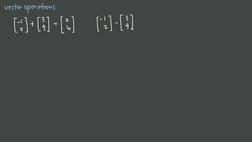PLAY PREVIEW

### Segments in this Video

#### Basic Operations of Vectors(04:09)

FREE PREVIEW

Vector addition is just a matrix addition. When adding vectors, we connect the initial point of the second vector to the terminal point of the first vector. And when subtracting vectors, we have to be careful with the order of the vectors.

To add vectors, connect the terminal point. When subtracting vectors, take the exact same vector and move it the opposite directions.

#### Multiplying Vectors (04:58)

The dot product is a way of multiplying two vectors together. It just takes the first component of each vector, and multiplies them together, and then adds the product of the second components.

#### Credits(00:00)

Credits

For additional digital leasing and purchase options contact a media consultant at 800-257-5126
(press option 3) or sales@films.com.

# Vector operations

Part of the Series : Integral Calc: Linear Algebra
 3-Year Streaming Price: \$49.95

Share

### Description

This video tutorial works through math problems/equations that address topics in Linear Algebra. This specific tutorial addresses Vector operations.

Length: 11 minutes

Item#: FMK275901Closed Captioned